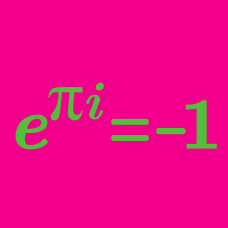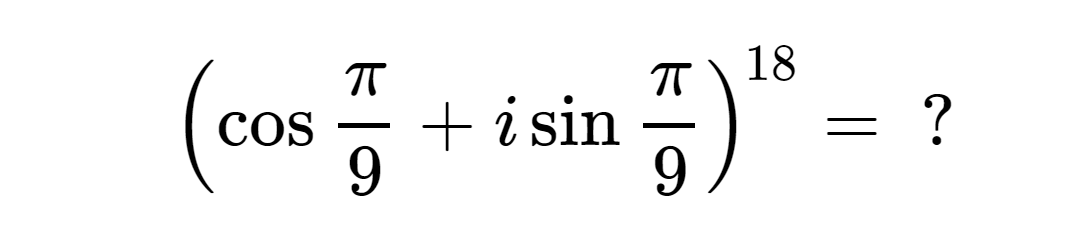Algebra

# De Moivre's Theorem - Raising to a PowerEvaluate $\left(\frac{\sqrt{2}+i\sqrt{2}}{2}\right)^{64}.$

Let $z$ be a complex number such that $z = \sqrt{2}(\cos 4 ^{\circ} + i \sin 4 ^{\circ}).$ Then $z^{8}$ can be expressed as $r( \cos \alpha^{\circ} + i \sin \alpha^{\circ})$, where $r$ is a real number and $0 \leq \alpha \leq 90$. What is the value of $r+\alpha ?$

Details and assumptions

$i$ is the imaginary number such that $i^2=-1$.

What is the value of $\left( \cos \frac{\pi}{8} + i \sin \frac{\pi}{8} \right)^{16}$?

Details and assumptions

$i$ is the imaginary unit, where $i^2=-1$.

If \begin{aligned} x & =\cos \frac{\pi}{14}+i\sin \frac{\pi}{14},\\ y &= \cos \frac{9}{14}\pi+i\sin \frac{9}{14}\pi, \end{aligned} what is the value of $x^{5} y^{15}$?

×